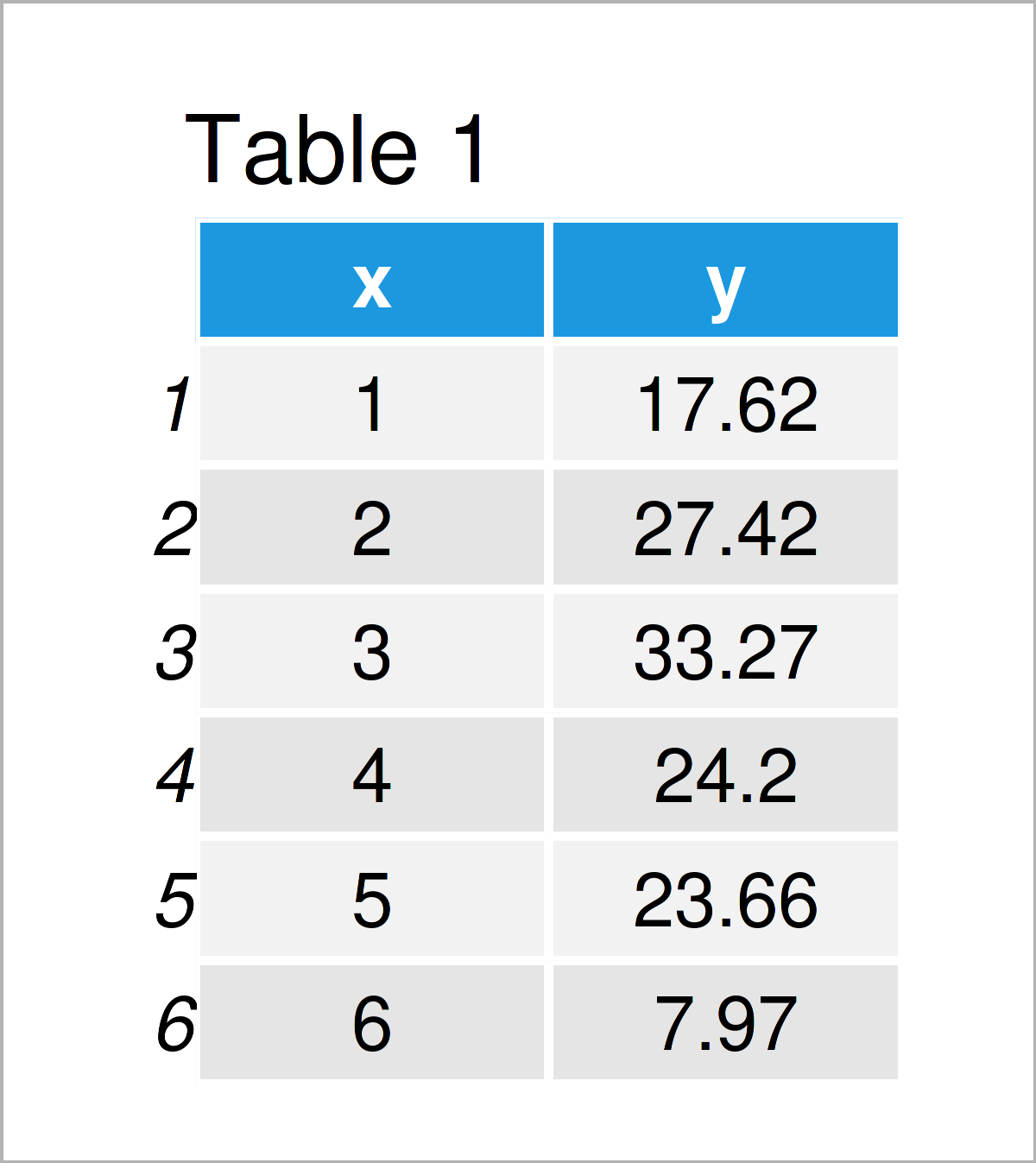# Get Equation of Linear Trend Line in R (Example)

In this R tutorial you’ll learn how to extract the equation of a linear regression line.

So now the part you have been waiting for – the example!

## Creation of Example Data

First, we need to create some data that we can use in the examples below:

```set.seed(238679)                   # Create example data
data <- data.frame(x = 1:100,
y = round(1:100 + rnorm(100, 10, 10), 2))Have a look at the previous table. It shows the first six rows of our example data, and that our data is composed of the two columns “x” and “y”.

Next, we can draw our data:

```plot(data\$x,                       # Draw line plot of data
data\$y,
type = "l")```After running the previous R programming code the line plot shown in Figure 1 has been created.

## Example: Extract Equation of Linear Regression Line

This example shows how to get the equation of the time trend shown in Figure 1. For this, we first have to estimate a linear regression model:

`my_mod <- lm(y ~ x, data)          # Estimate linear regression model`

We can visualize the output of our linear regression by adding the regression line to our line plot:

```plot(data\$x,                       # Draw line plot with regression line
data\$y,
type = "l")
lines(data\$x,
predict(my_mod),
col = 2,
lwd = 2)```The output of the previous R code is shown in Figure 2 – We have created a line trend plot with a straight regression line.

Next, we can extract the coefficients of our model using the coef function:

```my_coef <- coef(my_mod)            # Extract coefficients of model
my_coef                            # Print coefficients of model
# (Intercept)           x
#  12.2356788   0.9764044```

The previous output shows the intercept and the regression coefficient of our variable x.

Next, we can use these model estimates to print our equation using the paste function:

```my_equation <- paste("y =",        # Extract equation of model
coef(my_mod)[],
"+",
coef(my_mod)[],
"* x")
my_equation                        # Print equation of model
#  "y = 12.2356787878787 + 0.976404380438045 * x"```

Have a look at the previous output: It shows the equation of the linear regression line that we have estimated before.

## Video & Further Resources

I have recently published a video on my YouTube channel, which shows the R syntax of this article. Please find the video below.

Please accept YouTube cookies to play this video. By accepting you will be accessing content from YouTube, a service provided by an external third party.If you accept this notice, your choice will be saved and the page will refresh.

Furthermore, you might want to have a look at some of the other tutorials on my website.

In summary: This article has demonstrated how to get the equation of a linear regression slope in R programming. In case you have additional questions, please let me know in the comments.

Subscribe to the Statistics Globe Newsletter

•Zulfiya
August 28, 2023 5:42 am

Hello! can I write this “12.2356787878787” in this format “12.23”
“digits” function not working

•August 28, 2023 8:19 am

Hello Zulfiya,

I think it’s better to use the round() function. Using the digits argument with the options() function would change the global settings. See the example with the round function below.

```number <- 12.2356787878787
formatted_number <- round(number, 2)
print(formatted_number)
#12.24```

Best,
Cansu

••August 28, 2023 8:54 am